﻿ LS coupling and magnetization of 4f rare-earth, 3d metals

Rare-earth and metals really show "Spin" ?

Alkali D lines = "Spin" - orbit coupling is wrong.

Potassium (= K ) D lines don't represent spin-orbit interaction. ← Too strong !

(Fig.1) Hydrogen and potassium fine structure (= D line ).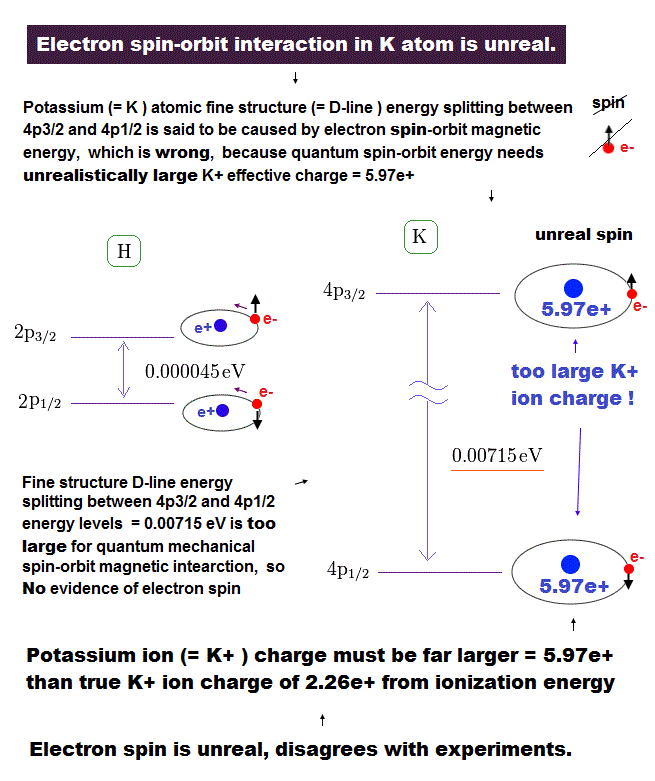On this page, we show sodium D-lines are too big to be explained by spin-orbit interactions.
It is quite natural that we think these unrealistically large effects in D lines are due to some Coulomb interactions with inner electrons.

On this page, we show spin-orbit interactions in all other alkali metals such as potassium (= K ), rubidium (= Rb ), and cesium (= Cs ) are unrealistically too big, which means the concept of spin - orbit coupling is just an illusion.

On this site, hydrogen fine structure (= doublet ) between 2p3/2 and 2p1/2 is about 0.000045 eV (= 0.4 T ).
The fine structure between 4p3/2 and 4p1/2 of potassium is as big as 0.00715 eV (= 63 T ), as shown on this site or this site (p.7, n.67).

D-lines are "too widely " separated to be explained by spin-orbit coupling.

The difference between hydrogen and potassium D-lines is great ( 0.4 T vs. 63 T ).
Approximately, we can consider the outer 4p ( or 4s ) electron of potassium is moving around Z = +1 central core charge.
( "Core" is the total charge of K nucleus and all electrons contained in n = 1, 2, 3 orbits. )

[ If potassium D lines are caused by spin-orbit coupling, Z becomes too big ( 1 → 5.97 ) ! ]

(Fig.2) Spin-orbit interaction ? → Central charge Z becomes "too big".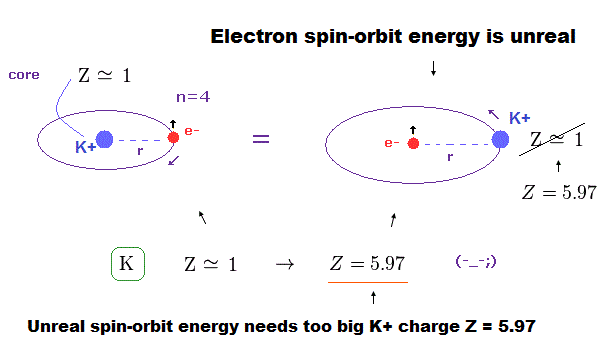From the viewpoint of this outer electron, the core charge is moving around in the opposite direction, which causes magnetic field at the point of the electron having "spin".
As a result, spin-orbit interaction is produced, they insist.

Effective core charge becomes Z = 5.97 (← too big ! ) in K !?

Both in hydrogen and potassium's outer (= 4p ) electron, the effective central (= core ) charges they feel should be about Z = +1e.
But the discrepancy between these H and K spin-orbital interactions are too wide.
This means the effective central charge in potassium is much bigger than Z = 1 (= about Z = 5.97 in K ! ), which is very unreasonable and proves "Spin" doesn't exist.

(Fig.3) Energy difference between p3/2 and p1/2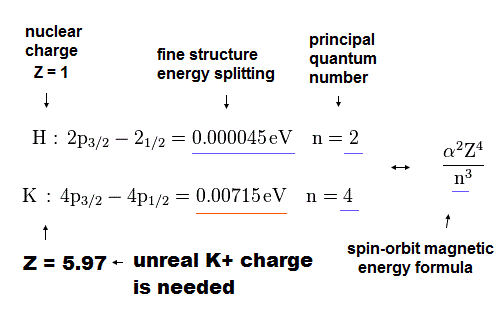According to this site (p.9) and this site (p.13), the spin orbit interactive energy is proportional to Z4 / n3.
( Z is central positive charge, n is principal quantum number, α is constant. )

In hydrogen atom, Z = 1 and n = 2.
In potassium atom, n =4.
From these values and energies ( 0.000045 eV vs. 0.00715 eV ), we can determine effective central charge Z of potassium.

Spin-orbit coupling in Potassium is false.

(Fig.4) Z of potassium is too big (= 5.97 ).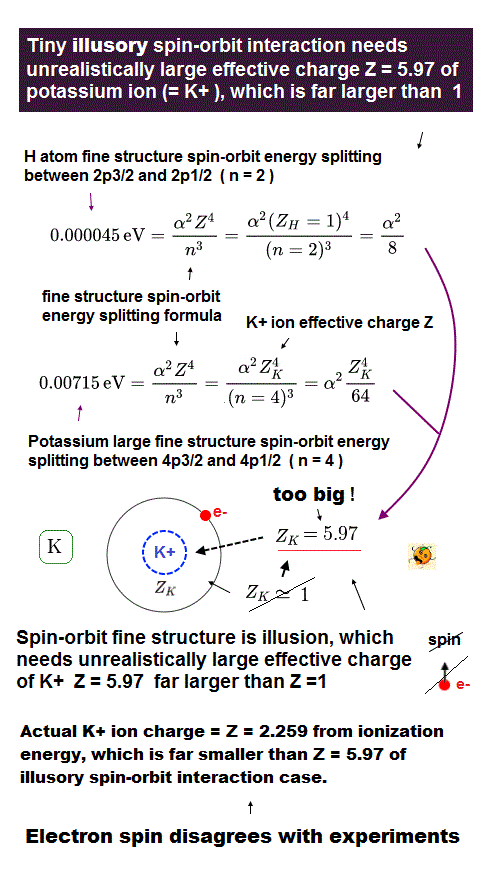From Fig.3 and Fig.4, the effective core charge of K becomes as large as Z = 5.97, which is unrealistic.
This core charge is the total charge summing up K nucleus and all 1n, 2n, 3n electrons.
The charge of K+ ion ( excluding only 4s electron ) is about +1e.
So, this core charge "Z" must be close to "1" also in K.

In conclusion, it is very unnatural that we consider the fine structure of potassium D lines is caused by spin-orbit interaction.
As far as I see various websites and textbooks, there are NO reasonable reason why the K fine structure is much bigger than H.

Coulomb ( NOT spin-orbit ) interaction causes D lines.

(Fig.5) Coulomb and large (core) orbit - orbit interactions generate "K" D lines.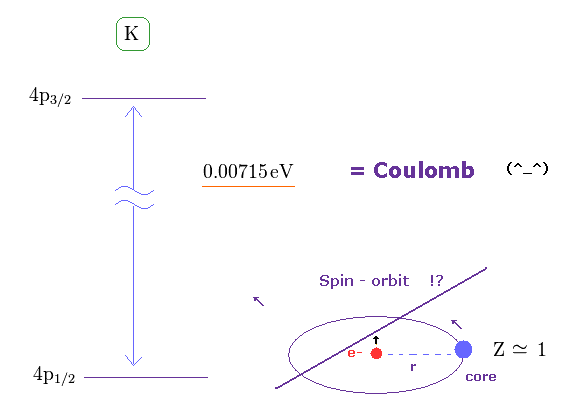In the present Lange g factor, they insist there are No magnetic moments in the inner (= core ) electrons in K.
But from the realistic viewpoint, the inner shells contain various large orbital motions.

So we can naturally think the various Coulomb interaction and large magnetic moment of inner electrons cause this large fine structure in potassium D lines.
Spin- orbit interaction is too weak to explain potassium D lines.

How big is effective core charge Z of "K" from ionization energy ?

(Fig.6) What is effective core charge Z from ionization energy ?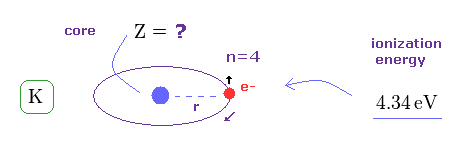In Fig.4, if spin-orbit interaction is real, effective core charge Z of potassium becomes much bigger (= 5.97 ) than "1".
We can know this effective core charge from ionization energy of potassium ( 4s ) outer electron.
This ionization energy of potassium is 4.34 eV.

(Fig.7) hydrogen-like atoms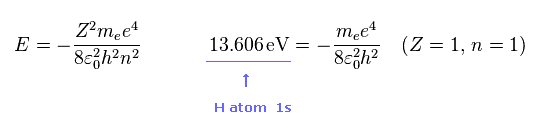Fig.7 is energy levels in each "n" and "Z" of hydrogen like atoms.
As you know, hydrogen ionization energy of 1s electron is 13.606 eV.
In 1s hydrogen, Z = 1 and n = 1.

True effective core charge is only Z = 2.259 ( NOT 5.97 ).

(Fig.8) True effective core charge is Z = 2.259 in potassium.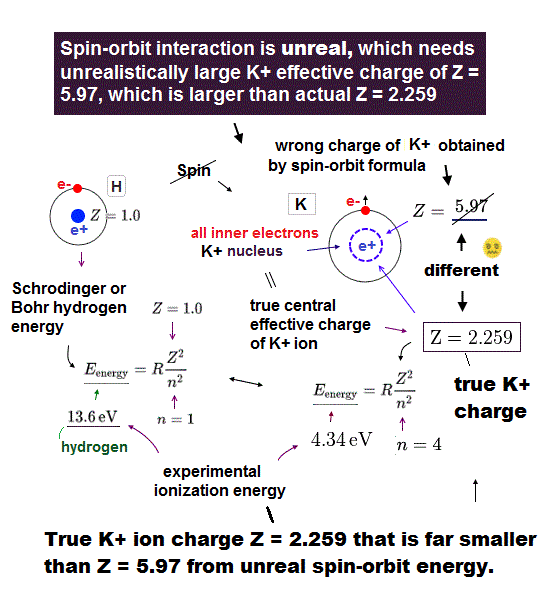Considering the principal quantum number ( n = 4 ), ionization energy (= 4.34 eV ) in "K" and Fig.7, we get the effective central charge Z = 2.259 in K 4s electron.

This true effective core charge (= 2.259 ) is much smaller than Z = 5.97 in Fig.4.
So the concept of spin-orbit coupling in potassium is self-contradictory and wrong.

Spin-orbit coupling in Rubidium (= Rb ) is false, too.

(Fig.9) Fine structure of Rb.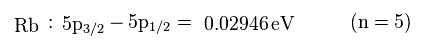As shown on this site, the energy difference between 5p3/2 and 5p1/2 in Rb is 0.02946 eV.
This fine structure of Rb is much bigger than hydrogen (= 0.000045 eV ), too.

(Fig.10) Effective central charge Z = 10.25 is too large.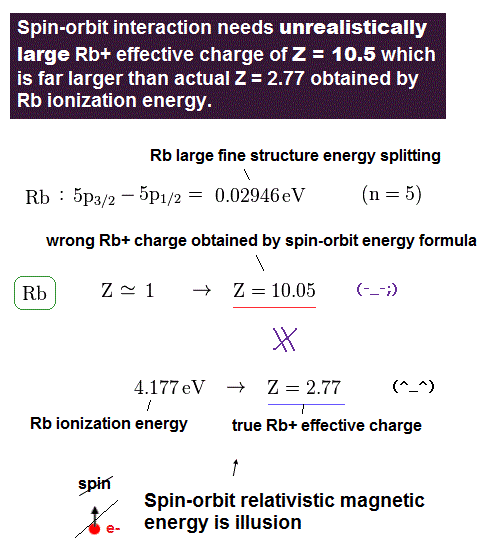Like potassium case, we can compute effective central charge based on spin-orbit and ionization energies.
Central charge based on spin-orbit becomes too large ( Z = 10.05 ) in comparison to true effective charge ( Z = 2.77 ).
This result shows spin-orbit interaction of Rubidium is wrong, too.

Spin-orbit coupling in cesium (= Cs ) is false, too.

(Fig.11) Fine structure of Cs.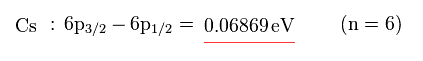As shown on this site, the energy difference between 6p3/2 and 6p1/2 in Cs is 0.06869 eV.
This fine structure of Cs is much bigger than hydrogen (= 0.000045 eV ), too.

(Fig.12) Effective central charge Z = 14.248 is too large.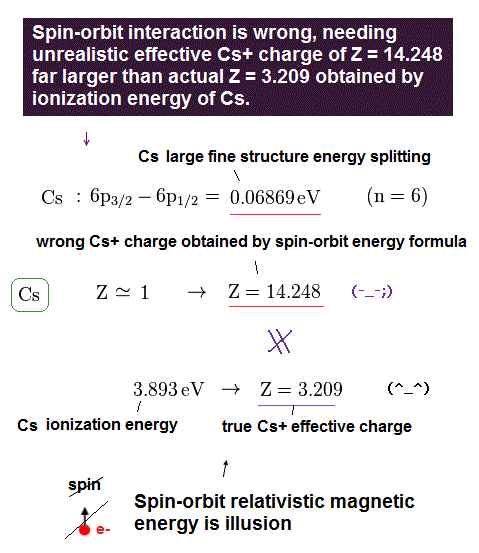Like potassium case, we can compute effective central charge based on spin-orbit and ionization energies.
Central charge based on spin-orbit becomes too large ( Z = 14.248 ) in comparison to true effective charge ( Z = 3.209 ).
This result shows spin-orbit interaction of cesium is wrong, too.

Rare-earth and 3d metals don't have "Spin".

[ "Artificial" rules for describing magnetization of metals. ]

(Fig.13) Magnetization of metals really proves spin-orbit (= LS ) coupling ?One of the main experimental results of strange Spin is magnetization of 4f rare-earth elements.
Magnetic property under some magnetic field should obey Lande g-factor ( see this site ) and LS coupling rules, according to quantum mechanics.

In fact, it is known that "3d" metals such as Ti, V, Cr, Mn, Co, Ni and Cu do NOT obey Lande g factor.
Their experimental grounding is Only 4f rare-earth ions.
All other elements show more complicated magnetic property, which cannot be described by simple Lande g factor.
( Even if you search for "effective Bohr magneton", only rare-earth ions can be found on the internet. )

Even in case of rare-earth ions, they introduced very artificial rules to fit them to experimental results.
So Spin-orbit interactions in various metals are also very doubtful and unrealistic.

3d metals don't satisfy Lande g factor. → Aritifical rule, L = 0 ?

(Fig.14) Angular momentums (= L ) of "3d" orbitals are all zero ? ← Wrong !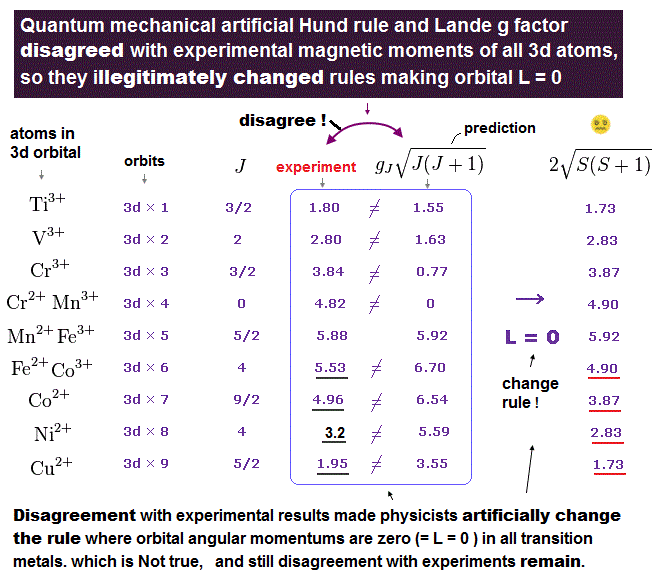According to the standard quantum mechanics, angular momentum of "3d" orbitals is L = 2, which is NOT zero.
But in "3d" metallic ions, the calculated resuts of spin-orbit coupling (+ Hund rule ) are completely different from the experimental values, if they have angular momentum.

To fit them to experimental data, they suddenly delete all angular mometum of 3d orbitals !
This is clearly artificial rule, and shows spin orbit couplings ( Hund rule, Lande g factor ) break down.

They insist transition-metallic ions are NOT independent from neighboring ions, and all of their orbital angular momentums are cancelled out. ( → L = 0 ).
But this interpretation is very forcible and convenient.
Only orbital magnetic moment becomes zero ? Though spin magnetic moment is completely intact. This is strange.

Unrealistic positions of " 4f " orbitals in rare-earth. ← Trick.

(Fig.15) "4f" orbitals are very far away from "4d" orbitals ! ← Aritificial tricks, again.Different from "3d" metals, it is known that magnetization of "4f" rare-earth ions is close to experimental results.
( See this site (p.425) or this site p.59. )
But in fact, they adopt some very artificial tricks in rare-earth elements.

One of the tricks is the positions of 4f orbitals in the periodic table.
As shown in Fig.15, higher energy levels such as 5s, 5p, 5d, and 6s appear before 4f orbitals !
In palladium (= Pd ), all of 4d orbitals are already occupied, but we have to wait for "4f" orbital to appear until "Ce" !

Strange orbitals of rare earth ions.

(Fig.16) Ionization : Nd → Nd3+.   5s and 5p electrons are skipped ??Surprisingly, rare earh ions obey very unreasonable rules, as shown in Fig.16.
In the ionization from Nd to Nd3+, three electrons in 6s and 4f electrons are removed, though 5s and 5p electrons are completely intact.

6s orbitals are lower than 4f orbitals with respect to energy !?
( 6s orbitals appear before 4f orbitals in Fig.15. )
This unrealistic jump to "6s" orbital is indispensable for describing Cs D lines.

They insist 5s and 5p orbitals exist outside of 4f orbital.
If so, Nd3+ ion should remove 5p electrons instead of 4f.
This is clearly artificial trick.

(Fig.17)   J = L -S or J = L + S ?? ← Trick.As I explain later, they apply different rules to small and large 4f metals.
The upper case of Fig.17 uses the equation of J = L - S.
The lower case of Fig.17 uses the equation of J = L + S.

Though directions of angular momentum and spin are the same in them, they intentionally change the sign of them.
As far as I check various other atoms and ions, this ad-hoc rule was introduced ONLY for rare-earth ions.
( Because pure effective Bohr magneton is valid ONLY in 4f rare-earth ions. )

This site (table 4) show, in other orbitals such as 3d, 4d, 5d, 5f excluing ONLY "4f", the influences by ligand-field become too strong to be neglected.
This means all these orbitals disobey simple Lande g factor.

Effective Bohr magneton number.

(Eq.1)Eq.1 is magnetic moment of some atom (ion).
μB is Bohr magneton, which magnitude is as same as Bohr's ground state orbit.
"J" is total angular momentum combining L and S.

Lande g factor in multi-electrons ?

(Eq.2) gJ = Lande g factor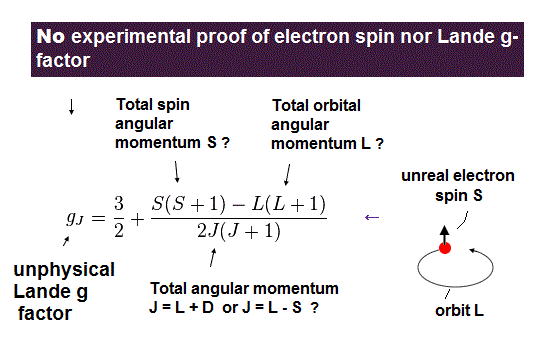As shown on this page, Lande g factor (= gJ ) is based on very unnatural precessions.
In multi-electron atoms, we need to sum up each spin like Eq.2, they insist.

The magnetic moments themselves are the same in spin and orbital angular momentums.
But in LS coupling, they consider "S" and "L" have completely different properties. This is strange.
( So, they clearly separate spin and orbital like S = S1 + S2 + S3 .. , L = L1 + L2 + L3 .. )

Energy = magnetic moment × magnetic field.

(Eq.3) E = magnetic energy.Under some external magnetic field H, the magnetic energy E is given by magnetic moment (= μJ ) × H.
MJ is the component of J in the direction of H.

M = average magnetic moment.

(Eq.4)The probability of some energy state is given by Boltzmann factor.
So the average magnetic moment M becomes like Eq.4.
B(x) is called "Brillouin function".

(Eq.5)To get effective Bohr magneton, we consider the magnetic moment in the limit that magnetic field H becomes zero ( H → 0 ).
Using Eq.5, Eq.4 becomes

Effective Bohr magneton in H → 0 limit is very doubtful.

(Eq.6) Effective Bohr magneton in the limit of H → 0.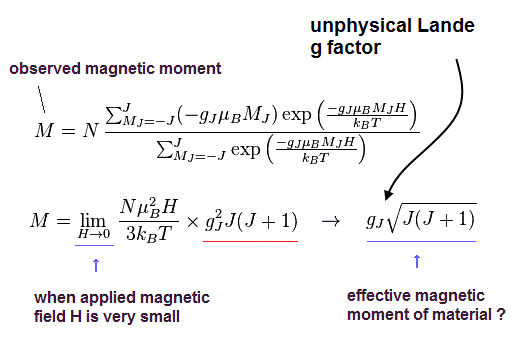In the limit of H → 0, we can get the effective Bohr magneton, which includes a square root of J(J+1).
( You need to remember this J(J+1) is gotten based purely on classical concepts such as Boltzmann factor, here. )

The important point is that it is extremely difficult to measure magnetization in the limit of H → 0.
Because various noises and thermal fluctuation cannot be neglected under very weak H.
So this effective Bohr magneton needs to be obtained by extrapolation based on data of H > 0.

"True" magnetic moment is an integer times Bohr magneton.

(Eq.7) True magnetic moment.When the external magnetic field H is strong enough, magnetization M of metals becomes like Eq.7.
Eq.7 doesn't contain a square root of J(J+1).
This value of Eq.7 under enough H is more reliable than Eq.6 of very weak H.

Magnetization under enough H is more believable than H → 0 limit.

(Fig.18) Effective Bohr magneton in the limit of H → 0 can be believed ?As shown on this site (p.422), magetizations of various metals ( ions ) tend to be an integer times Bohr magneton (= μB ) under some enough H.
This result indicates some Bohr's quantization rule is valid also in these metals.

On the other hand, effective Bohr magneton including a square root of J(J+1) is obtained from the slope of magnetization curve in the limit of H → 0.
So these results are very susceptible to various other effects, and we need to evaluate these results very carefully.

" 3d " transition metals do NOT show spin orbit coupling.

[ They suddenly "delete" every angular momentum. But spin is intact. Why ? ]

(Fig.19) Lande g factor is invalid → angular momentum L = 0 !?As I said, all "3d" transition metallic ions do NOT satisfy Lande g factor and LS coupling rule.
They suddenly make every angular momentum zero ( L = 0 ) to fit them to experimental results.

This is very unreasonable.
Only magnetic moments caused by spin ( NOT orbital ) are left.
How can the law of nature distinguish magnetic moments caused by orbital mortion and spin ?
It's impossible.

They make angular momentum zero, by force. ← artificial tricks.

(Fig.20) Vanadium ion ( V3+ ) : L = 3 → L = 0 !?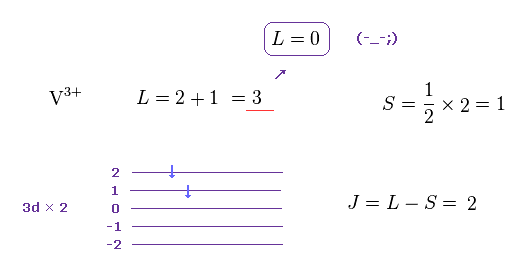For example, vanadium ion ( V3+ ) has two outer electrons in 3d orbitals.
According to Hund rule ( see this site ), the orbital angular momentum (= L ) of V3+ becomes 2+1 = 3.

And total spin angular momentum is S = 1.
Though "3d" orbitals clearly possess orbital motion, they suddenly delete them ( L = 3 → L = 0 ).
This trick is clearly unacceptable.

How can the surrounding enviroment distinguish spin and orbital magnetic moments, conveniently ?
This mechanism is too good to be true. Impossible.

All metallic ions satisfy an integral multible of Bohr magneton.

(Fig.21) Magnetic moment of V3+ ion becomes just 2.0 × Bohr magneton (= μB ).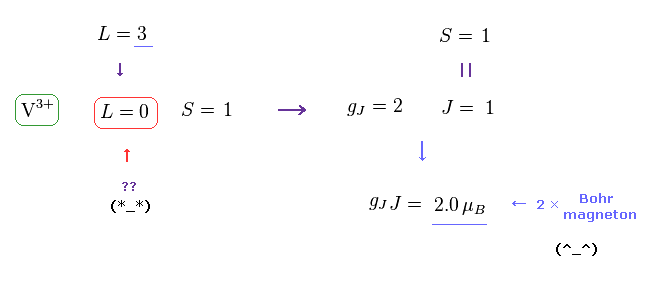If angular momentum (= L ) is zero, and only spin is left, Lande g factor becomes extremely simple ( gJ = 2 ).
And total angular momentum J is equal to S ( J = S ).

In V3+, saturation magnetization (= gJJ, plateau line of Fig.18 ) becomes just 2.0 × Bohr magneton, which means Bohr-Sommerfeld quantization rule is valid in all 3d metals.

(Fig.22) Bohr quantization rule is valid in all 3d metallic ions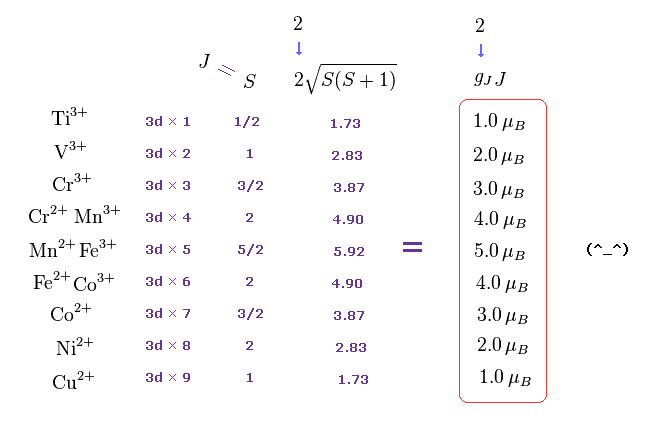A square root of S(S+1) is fictitious precession state, which cannot be directly confirmed.
The expeimental values (= gJJ ) which we can confirm are all a integer times Bohr magneton (= μB ), as shown in Fig.22.
So, Bohr-Sommerfeld quantization rule is valid in these ions.

An integer times Bohr magneton is shown, under enough H.

(Fig.18) Saturation magnetization (= gJJ ) under enough H.Neutral "3d" metals show much more complicated magnetic propertoes influenced by other surrounding atoms.
These neutral metals do NOT satisfy simple Lande g factor, and LS coupling.

It is known that these localization theories don't hold in neutral transition metals.
They need completely different theories.

Rare-earth ions depend on artificial tricks.

(Fig.23) Rare-earth ions really obey LS coupling ?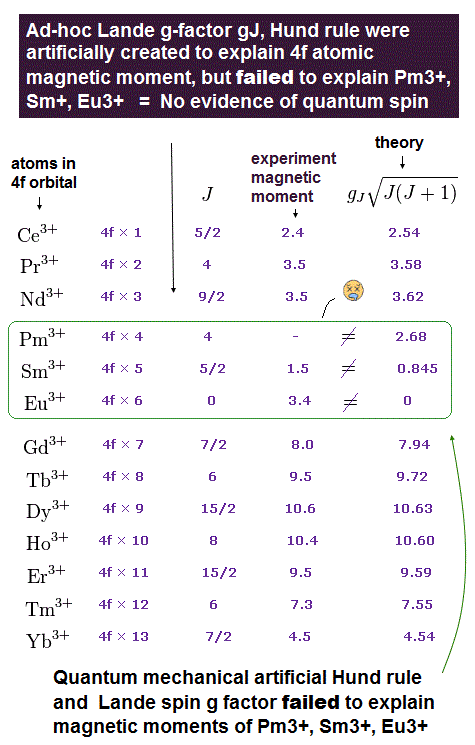Different from 3d transition metallic ions, "4f" rare earth ions are known to almost agree with LS coupling rule.
"gJ" is Lande g factor, which can be gotten from Eq.2.

But as I said, they depend on various ad-hoc rules to fit them to experimental results ( see Fig.15, 16, 17 ).
In spite of these ad-hoc rules, three rare-earth ions still do NOT obey Lande g factor, as shown in Fig.23.
These three elements expose the limit of these artificial tricks.

Rare-earth ions almost satisfy Bohr's quantization rule.

(Fig.24) "gJJ" becomes an integer times Bohr magneton.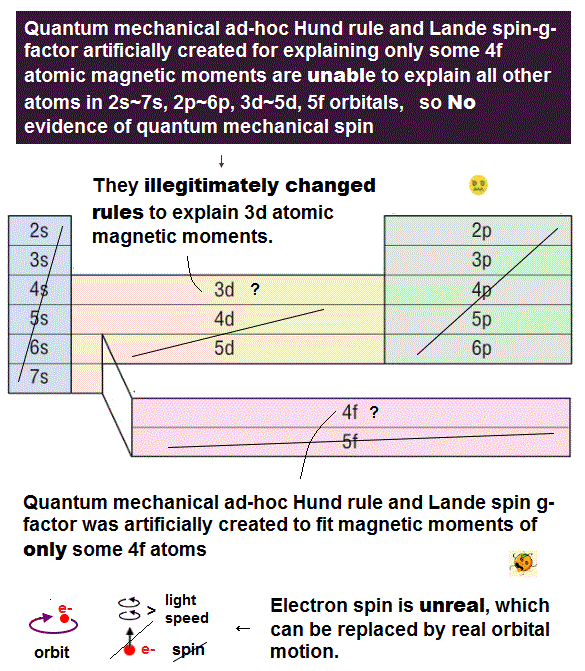Almost all textbooks tend to show data as effective Bohr magneton number including a square root of J(J+1).
But these states are fictitious precession states, which cannot be confirmed directly.
Saturation magnetization (= gJJ, plateau line of Fig.18 ) represents true magnetic properties.Suprisingly, almost all rare-earth ions obey Bohr's quantization rule with respect to gJJ, as shown in Fig.24.
These results indicate quantization of de Broglie waves play a important role in all atoms and ions.

Most artificial trick = "4f" rare-earth positions in Periodic table.

(Fig.25) Positions of "4f" rare-eath are very far away from 4d atoms !As you notice, the positions of "4f" rare-earth elements were very artificially determined to fit them to experimental results.
In Pd atom, all of 4d orbitals have already been filled (= 4d × 10 ).

Various higher energy levels such as 5s, 5p, 6s, 5d appear before "4f" orbitals.

"6s" orbitals are lower than "4f" orbitals !? ← Trick.

(Fig.26) Ionization : Nd → Nd3+.   5s and 5p electrons are skipped ??Strange to say, the present quantum mechanics insists the energy "6s" orbital is lower than that of "4f" orbital.
( "4d" < 5s < 5p < 6s < 5d < "4f". ← Trick ! )

This unrealistic positions of "6s" orbitals are indispensable for explaining Cs D lines.
The skipping n= 4, 5 orbitals (= 4f, 5d, 5f, 5g ) and jumping to 6s are very unreasonable and unacceptable.

Other Tricks.   J = L - S !?

(Fig.27) Which is true, J = L - S or J = L + S ?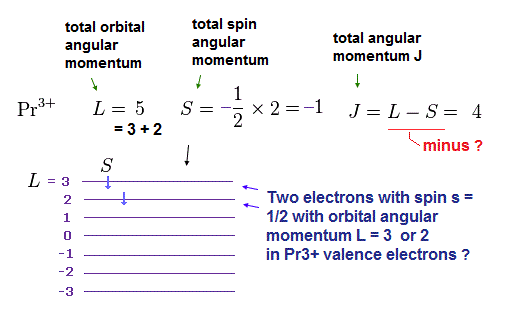Pr3+ ( praseodymium ion ) has two electrons in 4f orbitals.
According to basic Hund rule, orbital (= L ) and spin (= S ) angular momentums become "5" and "1", respectively, as shown in Fig.27.

The important point is that almost half of "4f" metals should satisfy J = L - S instead of J = L + S.
As far as I check various sites, this ad-hoc rule was introduced ONLY for explaining the experimental results of these rare-earth ions. See also this site.

J = L -S   → J = L + S   Artificial trick !

(Fig.28) Dysprosium ion ( DY3+ ) , J = L + S !?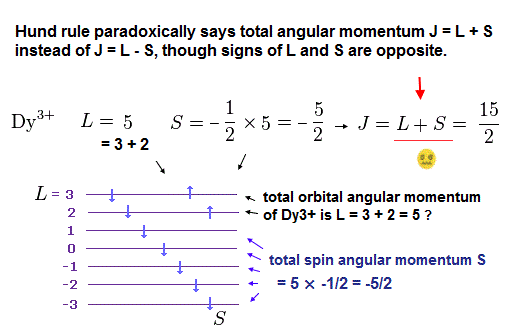On the other hand, in Dy3+ ion, they use the different equation of J = L + S.
This distinction is very unreasonable.

As shown in Fig.27 and Fig.28, the directions of each orbital and spin do NOT change.
So they should use the common formula J = L + S ( or L - S ).

(Fig.29) Directions are unchanged → J = L ± S is artificial trick.As shown in Fig.29', all spin directions are suddenly reversed, the instant one electron is added to 4f orbitals from Gd3+ to Tb3+.
This rule is too good to be true.

All spin directions are reversed !?   Impossible !

(Fig.29') All spin directions are reversed from Gd3+ to Tb3+ ??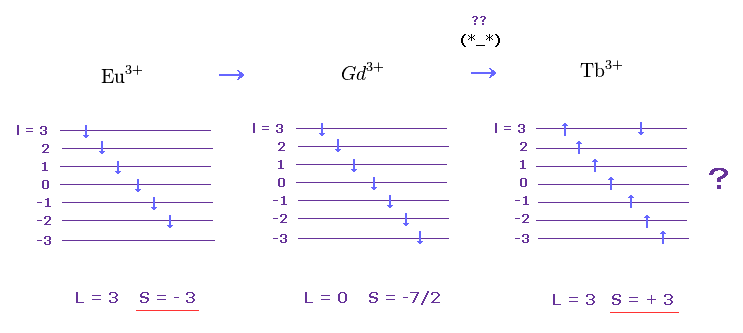This rule is valid ONLY in 4f rare-earth ions, which means it was introduced ONLY for 4f metals.
For example, "3d" transition metals do NOT obey LS couplicg rule, as I said in Fig.19.

This site (table 4) show, in other orbitals such as 3d, 4d, 5d, 5f excluding ONLY "4f", the influences by ligand-field become too strong to be neglected.
This means almost all orbitals disobey simple Lande g factor and Hund rule.

Hund's rules were created Mainly for rare earth.

On page 358, Physics 1971-1980 by Stig Lundqvist
-----------------------------------------------------
In 1925 Hund wrote a paper on the magnetic susceptibilities of rare earth compounds which was the crowning achievement of the empiricism ...
He utilized Lande's g factor and Hund rule .....
--------------------------------------------------
And in 1932, Van Vleck extended his theory to Eu and Sm.

Bohr's quantization rule is almost valid in rare-earth ions.

(Fig.30) Saturation magnetization = gJ J.As I said, effective Bohr magneton including a square root of J(J+1) is fictitious precession states, which cannot be confirmed directly.
( Only in the limit that external magnetic field H is zero, this relation appears . )

(Fig.31) Effective Bohr magneton in the limit of H → 0 can be believed ?Of course, when the magnetic field H is very weak ( H → 0 ), various noises cause errors.
If we consider saturation magnetization (= gJJ = plateau line of Fig.31 ), you will find rare earth magnetization is also close to an integer multiple of Bohr magneton (= μB ).

[ Examples. ]

(Fig.32) Cerium ion ( Ce3+ )(Fig.33) Pr3+ and Nd3+ ions.As shown in Fig.32 and Fig.33, saturation magnetizations (= gJJ ) of Ce3+, Pr3+ and Nd3+ are all close to an integer multiple of Bohr magneton.

(Fig.34) These ions are just an integer multiple of Bohr magneton.The cases of Tb3+, Dy3+, Ho3+ and Er3+ are very remarkable.
They are just an integer times Bohr magneton.
So you will find Bohr's quantization rule is almost valid even in these multi-electron rare-earth metals.

[ How about ions, which magnetizations are inconsistent with Lande g factor ? ]

(Fig.35)As shown in Fig.23, Lande g factor and LS coupling rule are completely violated in Eu3+ and Sm3+.
( They artificially try to introduce other effects to correct this discrepancy. But they aren't natural results at all. )

If we suppose total angular momentum of Eu3+ outer electrons are just "3", gJ becomes just "1".
As a result, gJJ becomes just 3.0 μB.

(Fig.36)In the same way, if we suppose total angular momentum of Sm3+ outer electrons are just "1.0", gJ becomes just "1.0".
As a result, gJJ becomes just 1.0 μB.

(Fig.37) "gJJ" becomes an integer times Bohr magneton also in rare-earth ions.In Pm3+ ion, simple LS coupling rule breaks down.
They insist the data of Pm3+ magnetization is too difficult to be gotten.
But as you see Fig.37, the magnetization of Pm3+ propably becomes almost zero.
This is the reason why they cannot measure Pm3+ magnetization.

Even though they use various ad-hoc assumptions such as "4f" unnatural positions of periodic table and J = L - S, three rare-earth ions such as Pm3+, Sm3+, Eu3+ still do NOT satisfy LS coupling rule.
This result exposes the limit of these artificial tricks.2014/1/24 updated. Feel free to link to this site.